# Lexer vs Parser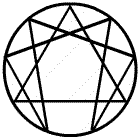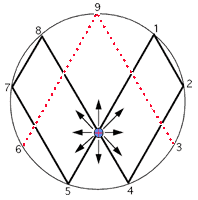Notice how the 3, 9, and 6 is in red and does not connect at the base. That is because it is a vector. The 1,2,4,8,7,5 is the third dimension while the oscillation between the 3 and 6 demonstrates the fourth dimension, which is the higher dimensional magnetic field of an electrical coil.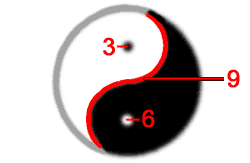The 3, 9, and 6 always occur together with the 9 as the control. In fact, the Yin/Yang is not a duality but rather a trinary. This is because the 3 and 6 represent each side of the Yin/Yang and the 9 is the "S" curve between them. Everything is based on thirds. We think that the universe is based on dualities because we see the effects not the cause.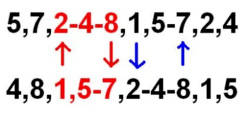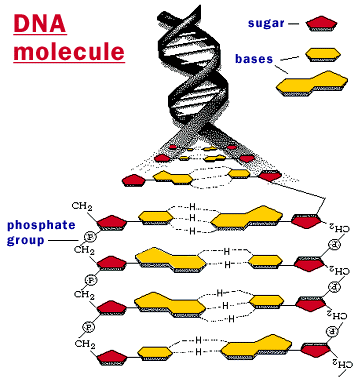### Four (4) Vector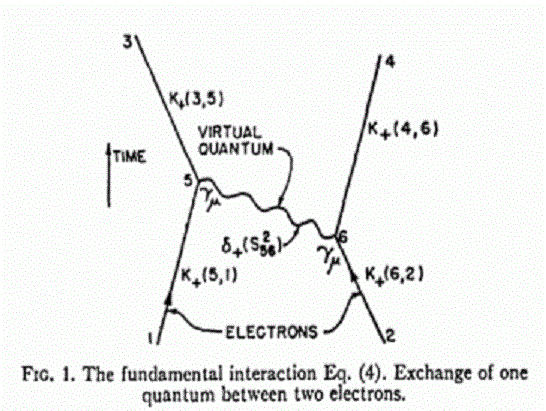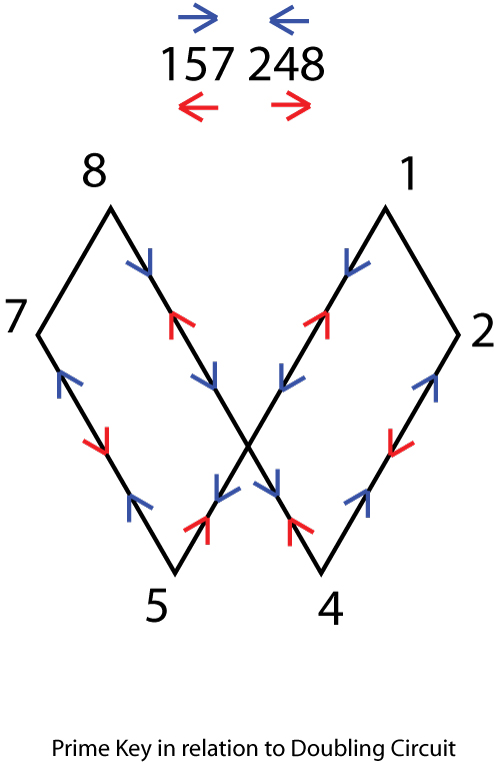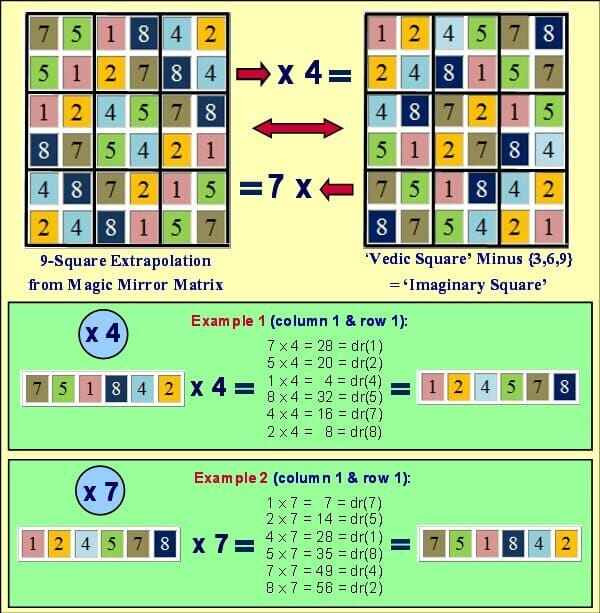!

Φ(11,13) = (114 - 10²) + 13 = 27

``````1729 = 7 x 13 x 19
1729 / 7 = 13 x 19 = 247

1729 = 7 x 13 x 19
7 + 13 = 20 = d(2)
└──  2 x 19 = 38

+----+----+----+----+----+----+----+----+----+----+----+----+----+----+
| {1}|  2 |  3 |  4 |  5 | {6}| {7}|  8 |  9 | 10 | 11 | 12 | 13 | 14 |
+----+----+----+----+----+----+----+----+----+----+----+----+----+----+
| {3}| {4}|  3 |  4 |  5 |  2 |  3 |  2 |  2 |  1 |  2 |  5 |  1 |  1 |{38}
+----+----+----+----+----+----+----+----+----+----+----+----+----+----+---- } 285
|  3 |  8 |  9 | 16 | 25 |{12}|{21}| 16 | 18 | 10 | 22 | 60 |{13}|{14}|{247}
+----+----+----+----+----+----+----+----+----+----+----+----+----+----+
|-- 38 ---|              |-- 33 ---|                        |-- {27}--|
``````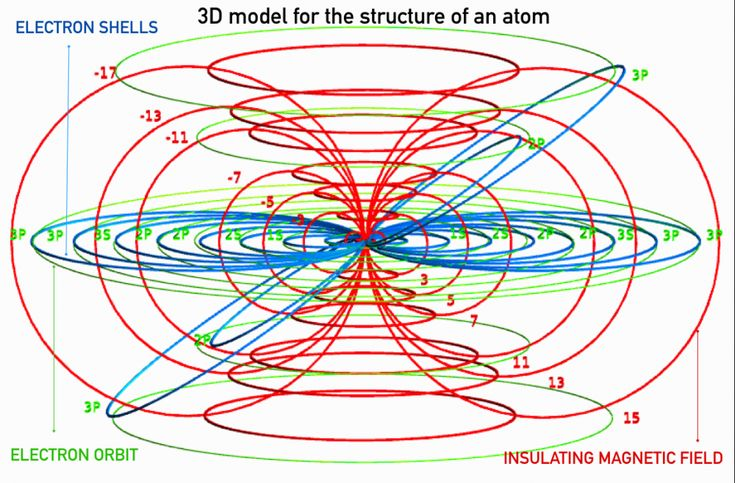``````------+------+-----+----------+-----+-----                                     |     ---
|      |     |     11:7 |   5 |   9 |  14 (20) --------› ¤               |      |
|      |  4  +----------+-----+-----+-----+                              |      |
|  3   |     |     12:8 |   9 |  60 |  40 | 109 (26) «------------       |     11¨  polymorphism
|      +-----+----------+-----+-----+-----+                       |      |      |
|      |     |     13:9 |   9 |  60 |  69 (27) «-- Δ19 (Rep Fork) | {2®} |      |
2   +------|  5  +----------+-----+-----+-----+                       |      |     ---
|      |     |    14:19 |   9 |  60 |  40 | 109 (28) -------------       |      |
|      |-----+----------+-----+-----+-----+                              |      |
|  4   |     | 15,18:11 |   1 |  30 |  40 | 71 (29,30,31,32) ------------      13¨  inheritance
329   |      |  6  +----------+-----+-----+-----+                                     |
|     |      |     |    19:12 |  10 |  60 | {70} (36) -------› Φ                      |
------+------+-----+----------+-----+-----+                                          ---
|      |     |    20:13 |  90 |  90 (38) ‹-------------- ¤                      |
|      |  7  +----------+-----+                                                 |
|  5   |     |       14 |            -----------------------------             17¨  class
|      |-----+----------+           |                             |             |
|      |     |       15 |           |       LEADING SCHEME        |             |
3   +------+  8  +----------+-----      |    (Multiplication Zone)    |            ---
|      |     |       16 |           |                             |             |
|      |-----+----------+-----+      -----------------------------              |
|  6   |     |    28:17 | 100 |                                                19¨  object
168   |      |  9  +----------+-----+                                                 |
|     |      |     |    29:18 | 50  | 50(68) ---------> Δ18                           |
------|------|-----+----------+-----+                                                ---
``````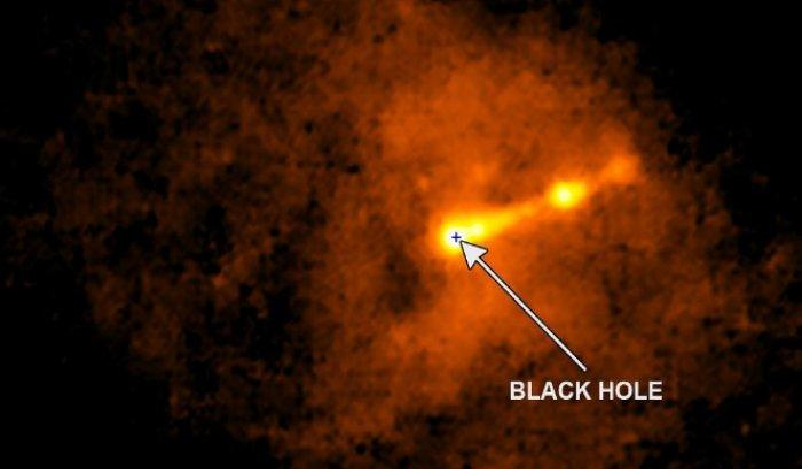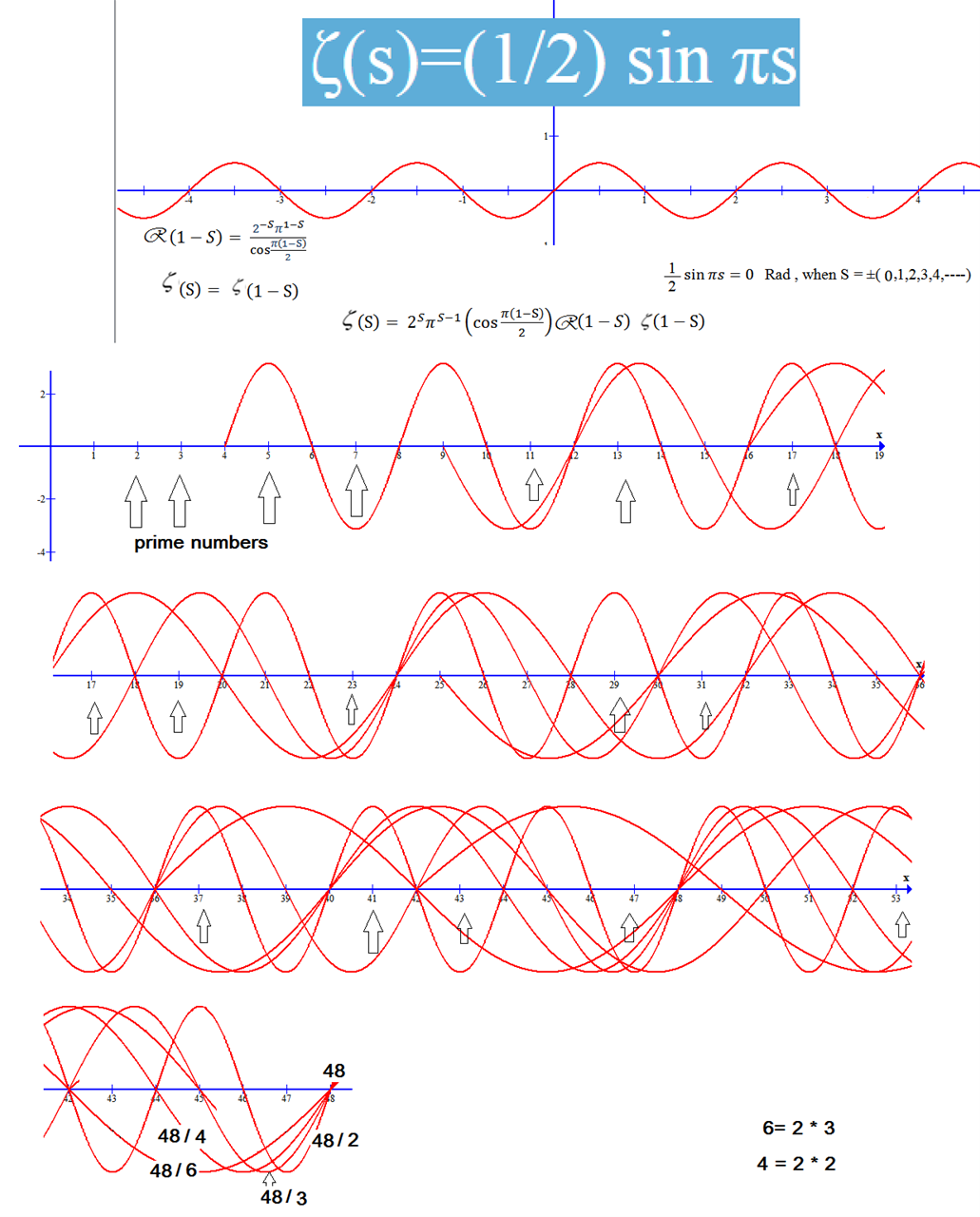With the above description then the instance configuration is via matrix integration from tensorflow which is placed at the end position to the turning point 100 vs 50 to the format (2,3) i.e. at id: 68.

π(1000) = π(Φ x 618) = 168 = 100 + 68 = (50x2) + (66+2) = 102 + 66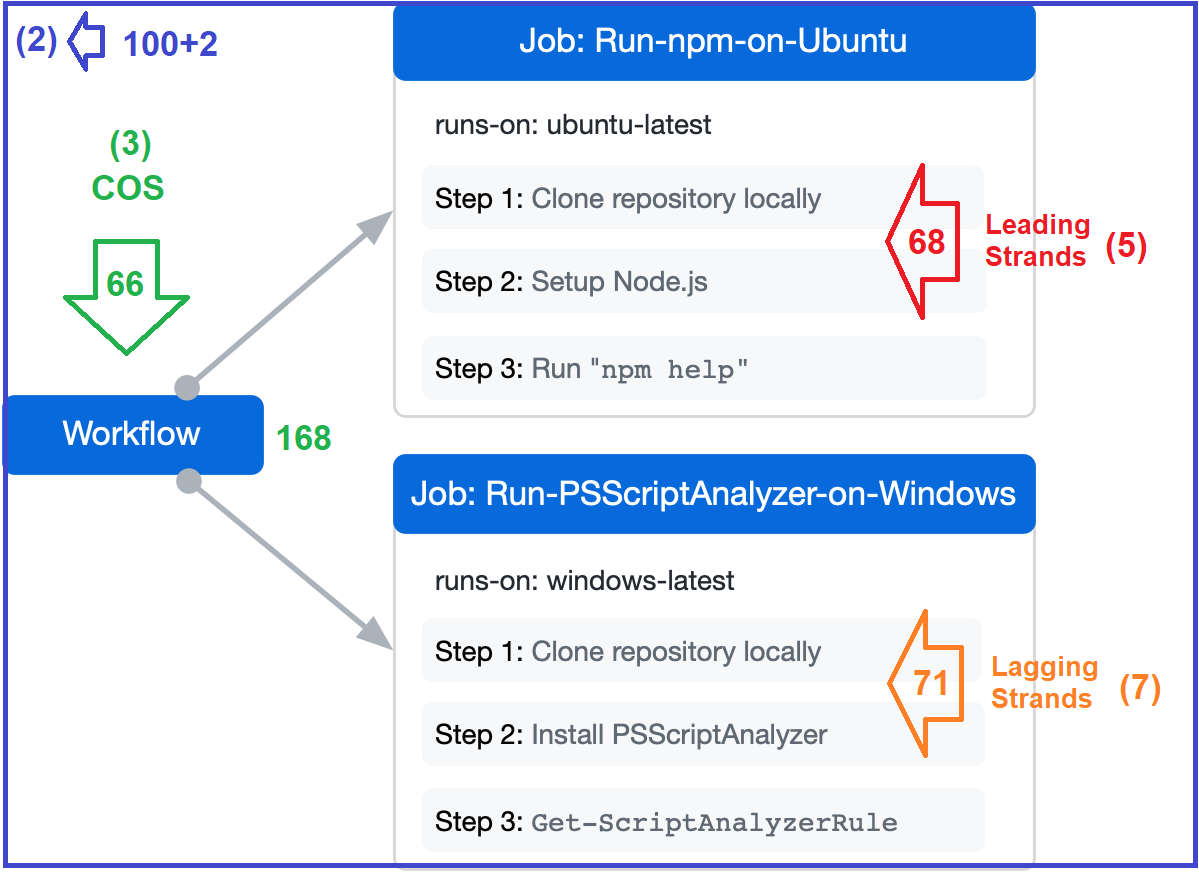30 + (5+13) + (7+11) + (17+19) = 30 + 18 + 18 + 36 = 30 + 36 + 36 = 102 = 2 + 100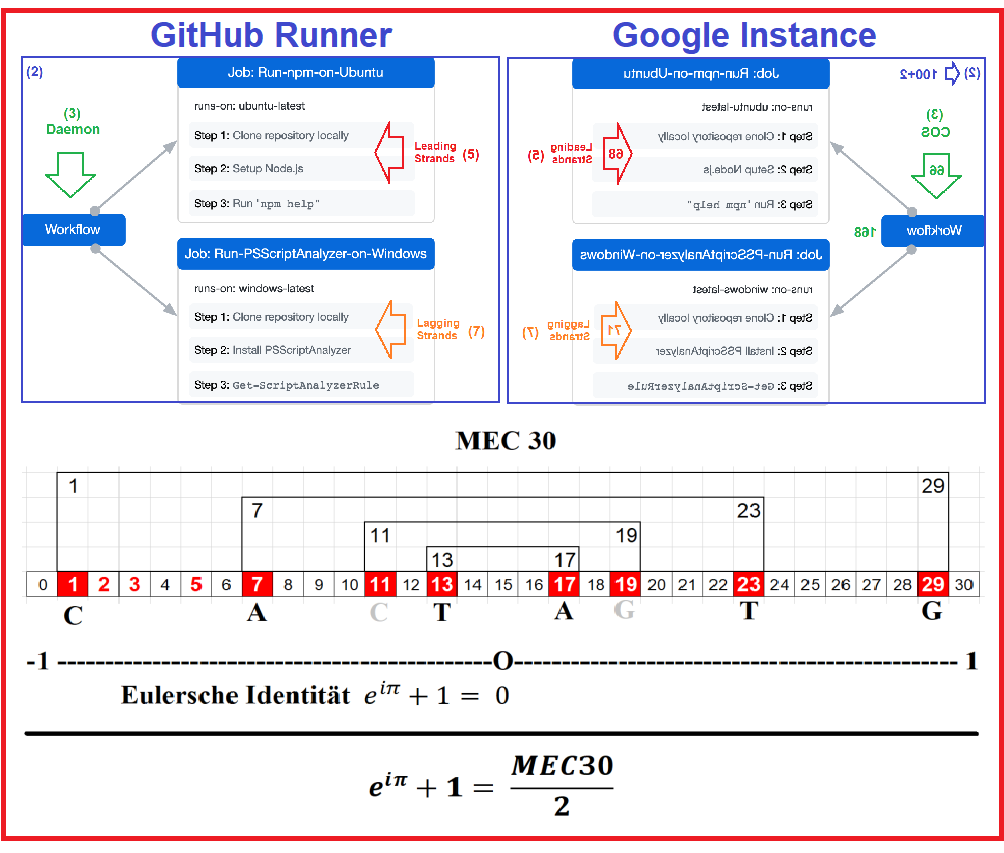With the above description, of course, you can guess where the next direction will be. That is from one (1) unit of DNA to one (1) unit of whole unity. That's why we need a solution of the remaining six (6) other cases to be placed with id: 11, 12, 14, 15, 26 and 28 so that id: 68 is congrued to the number two (2).Therefore the five (5) identities of (10, 11,12,14,15) are twisted prior joining the two (2) identities of (26,28). Since each of these seven (7) identities is linked by the eleven (11) objects then they turn to a strong seven (77). Lets's discuss them one by one.